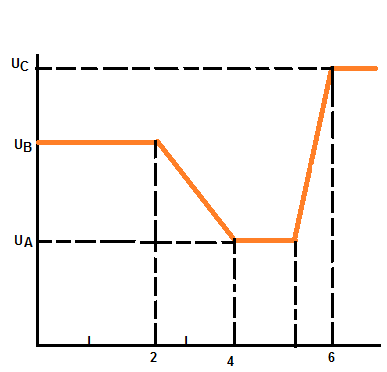# The figure here shows a plot of potential energy U versus position x of a 0.871 kg particle that...

## Question:

The figure here shows a plot of potential energy U versus position x of a {eq}0.871\ kg {/eq} particle that can travel only along an x axis. (Non conservative forces are not involved.) Three values are {eq}U_A = 15.0\ J,\ U_B = 35.0\ J {/eq} and {eq}U_C = 45.0\ J {/eq}. The particle is released at {eq}x = 4.50\ m {/eq} with an initial speed of {eq}7.74\ \dfrac m s {/eq}, headed in the negative x direction.

(a) If the particle can reach {eq}x = 1.00\ m {/eq}, what is its speed there, and if it cannot, what is its turning point? What are the

(b) magnitude and

(c) direction of the force on the particle as it begins to move to the left of {eq}x = 4.00\ m {/eq}?

Suppose, instead, the particle is headed in the positive x direction when it is released at {eq}x = 4.50\ m {/eq} at speed {eq}7.74\ \dfrac ms {/eq}.

(d) If the particle can reach {eq}x = 7.00\ m {/eq}, what is its speed there, and if it cannot, what is its turning point? What are the

(e) magnitude and

(f) direction of the force on the particle as it begins to move to the right of {eq}x = 5.00\ m {/eq}?## Conservative Force Field

For a conservative force field {eq}\vec{F} {/eq}, a scalar quantity called potential (potential energy) can be defined such that: {eq}\vec{F} \ = \ - \ \vec{\nabla V} {/eq}

Where: {eq}V {/eq} - is the potential

From work energy theorem, when a force {eq}\vec{F} {/eq} acting on a particle results in its displacement, then the work done by the force should equal change in kinetic energy of the particle i.e.

{eq}W \ = \ \int \vec{F}. \vec{dr} \ = \ {KE}_f \ - \ {KE}_i {/eq}

For a conservative force field, {eq}\int \vec{F} . \vec{dr} \ = \ V_i \ - \ V_f {/eq}

Therefore,

{eq}{KE}_i \ + \ V_i \ = \ {KE}_f \ - \ V_f {/eq}

Become a Study.com member to unlock this answer! Create your account

Given:

• Mass of the particle: {eq}m \ = \ 0.871 \ Kg {/eq}
• Values of potential energy:{eq}U_A \ = \ 15 \ J {/eq}, {eq}U_B \ = \ 35 \ J {/eq} and...Conservative Forces: Examples & Effects

from

Chapter 5 / Lesson 8
7.6K

Learn how to tell if a force is conservative and what exactly is being conserved. Then look at a couple of specific examples of forces to see how they are conservative.